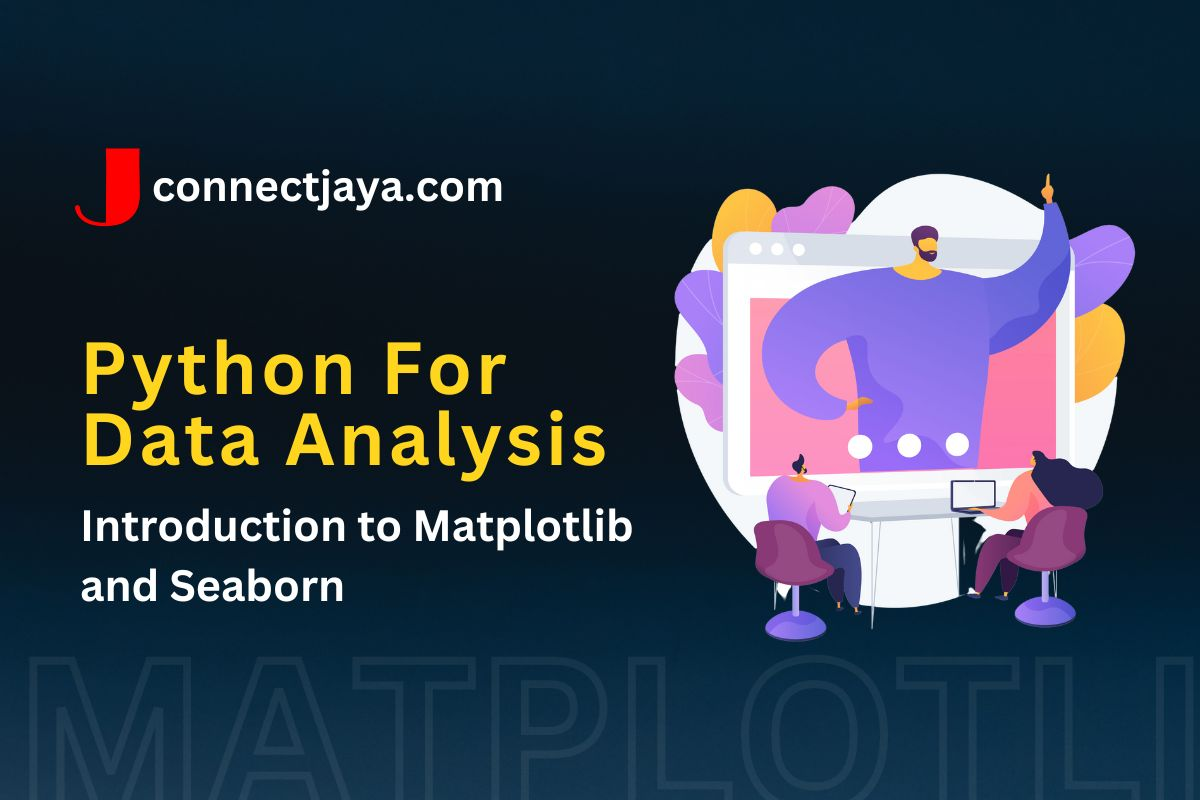## Introduction to Matplotlib and Seaborn

Matplotlib and Seaborn are two popular Python libraries for data visualization. Matplotlib is a low-level library that provides basic plotting functionality, while Seaborn is built on top of Matplotlib and provides more advanced plotting capabilities with a focus on statistical graphics. Here’s an introduction to both libraries:

### Matplotlib

import matplotlib.pyplot as plt

# create a simple line plot
x = [1, 2, 3, 4, 5]
y = [2, 4, 6, 8, 10]
plt.plot(x, y)
plt.xlabel('x-axis')
plt.ylabel('y-axis')
plt.title('Simple Line Plot')
plt.show()

# create a scatter plot with color-coded points
import numpy as np

x = np.random.randn(100)
y = np.random.randn(100)
colors = np.random.rand(100)
plt.scatter(x, y, c=colors)
plt.xlabel('x-axis')
plt.ylabel('y-axis')
plt.title('Scatter Plot with Color-Coded Points')
plt.show()


### Seaborn

import seaborn as sns

# create a histogram of a numerical variable
sns.histplot(df['quantity'])
plt.xlabel('Quantity')
plt.ylabel('Count')
plt.title('Histogram of Quantity')
plt.show()

# create a box plot of a numerical variable grouped by a categorical variable
sns.boxplot(x='product_type', y='price', data=df)
plt.xlabel('Product Type')
plt.ylabel('Price')
plt.title('Box Plot of Price by Product Type')
plt.show()

# create a scatter plot matrix of multiple numerical variables
sns.pairplot(df[['quantity', 'price', 'order_date']])
plt.show()


These are just a few examples of the many types of visualizations that can be created using Matplotlib and Seaborn. The specific types of plots used will depend on the characteristics of the data and the goals of the analysis.